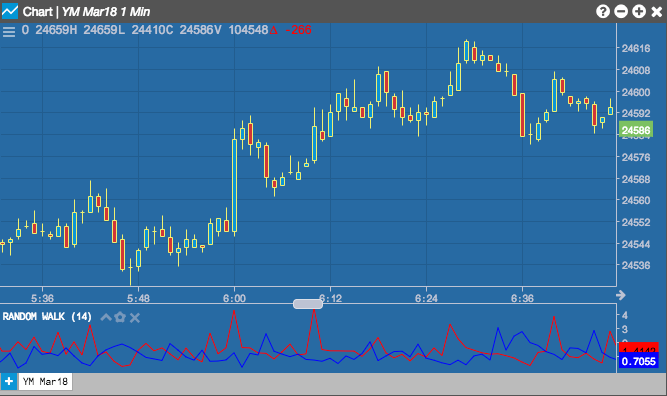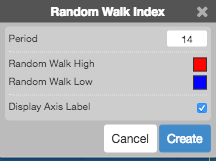Charts

# Random Walk Index

The Random Walk Index indicator determines if price movement is random or the result of a statistically significant trend over a specific time period.## Configuration Options• Field: Price or combination of prices to use as the base for average calculations. Possible values include:
• Open
• High
• Low
• Close
• HL/2 $$\left ( \frac{High + Low}{2} \right )$$
• HLC/3 $$\left ( \frac{High + Low + Close}{3} \right )$$
• HLCC/4 $$\left ( \frac{High + Low + Close + Close}{4} \right )$$
• OHLC/4 $$\left ( \frac{Open + High + Low + Close}{4} \right )$$
• Color Selectors: Colors to use for graph elements.
• Display Axis Label: Whether to display the most recent value on the Y axis.

## Formula

$RW_{low} = \frac{High_{1-n} - Low}{ATR_{n} * \sqrt n}$

$RW_{high} = \frac{High - Low_{1-n}}{ATR_{n} * \sqrt n}$

where ATR is the average true range over N periods preceding the current period.